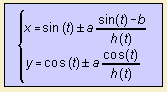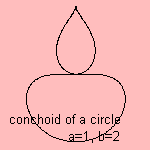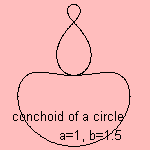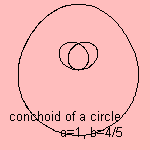# conchoid of a circle

## mainwith h(t) = √((sin(t)-b)2+cos2(t))

This is the conchoid of a circle, given a unity circle and a fixed point O(0, b) and a conchoid constant a.
When the constant a is equal to the radius of the circle (i.e. 1), five situations can be distinguished for the kind of curve:

• 0 < b < 1: one large eggform and two small ones
• b = 1: trisectrix
• 1 < b < 2: three parts
• b = 2: two parts
• b > 2: two parts and one singularity